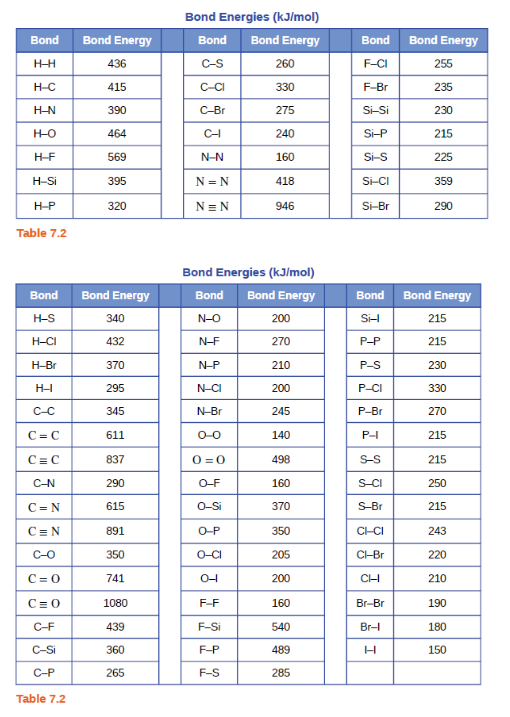# Problem: Using the bond energies in Table 7.2, determine the approximate enthalpy change for each of the following reactions:(b) H2C = CH2(g) + H2(g) ⟶ H3CCH3(g)

###### FREE Expert Solution
100% (369 ratings)
###### Problem Details

Using the bond energies in Table 7.2, determine the approximate enthalpy change for each of the following reactions:
(b) H2C = CH2(g) + H2(g) ⟶ H3CCH3(g)Frequently Asked Questions

What scientific concept do you need to know in order to solve this problem?

Our tutors have indicated that to solve this problem you will need to apply the Bond Energy concept. You can view video lessons to learn Bond Energy. Or if you need more Bond Energy practice, you can also practice Bond Energy practice problems.

What textbook is this problem found in?

Our data indicates that this problem or a close variation was asked in Chemistry - OpenStax 2015th Edition. You can also practice Chemistry - OpenStax 2015th Edition practice problems.